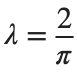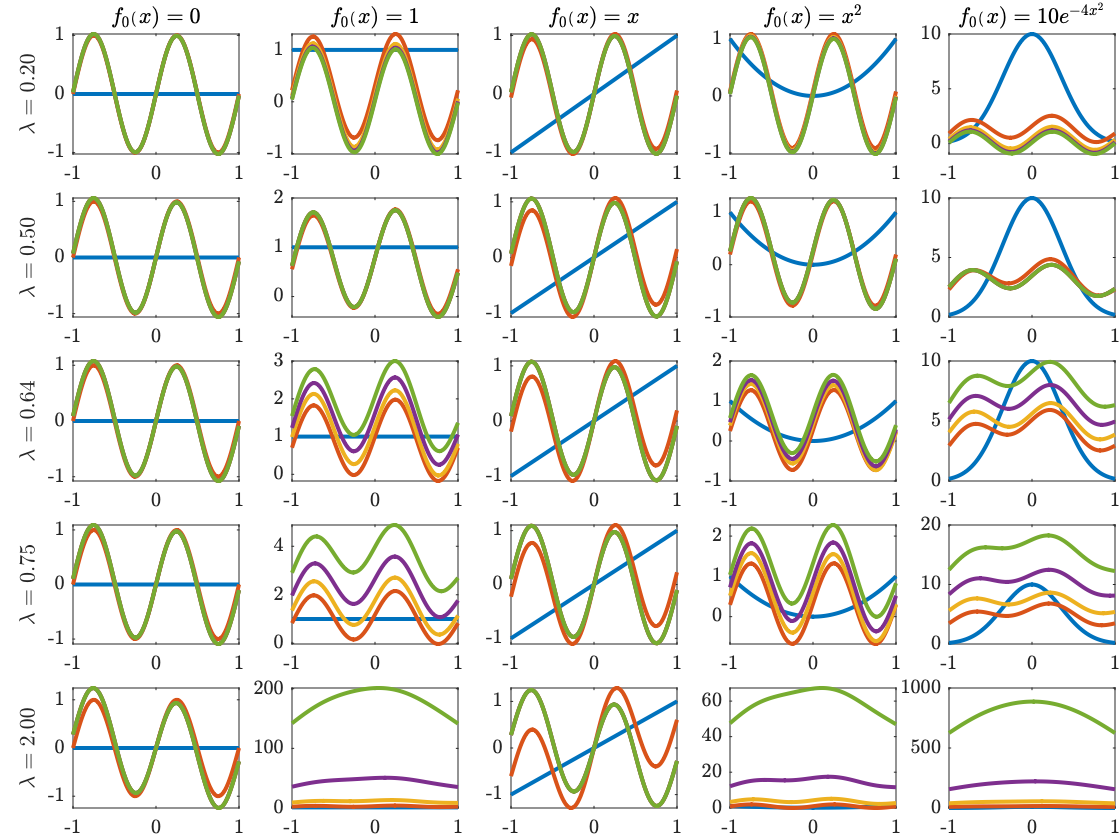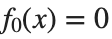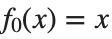# Patrick's solution to Meiss, Ch. 3, problem 4c

This solution makes use of three MATLAB features, the integral function that does integrals adaptively and numerically, anonymous functions, which allow you to pass user-defined functions into other functions, such as integral, and the arrayfun function, which evaluates the anonymous function fk at each point of the array x.
This is Patrick Grice's code, with some editing and live-script annotation by Prof. Goodman.
By using a Live Script, I have been able to explain my code a little bit better and include the outputs in a nice little report. I have chosen to export it to HTML which looks better. PDF export is also available, but it doesn't always break the pages in nice places.

#### First set up arrays of lambda values and functions

I chose five values of λ: the critical value for convergence, two above it, and two below it.
lambdas = [.2 .5 2/pi .75 2];
nLambda=length(lambdas);
f0s = {
@(x) 0, ...
@(x) 1, ...
@(x) x, ...
@(x) x.^2, ...
@(x) 10*exp(-4*x.^2) ...
};
f0string = {'$f_0(x)=0$',...
'$f_0(x)=1$',...
'$f_0(x)=x$',...
'$f_0(x)=x^2$',...
'$f_0(x)=10 e^{-4 x^2}$'};

nf0=length(f0s);
nIterates=4;
x = linspace(-1, 1, 101);

#### Set up an array of mini-plots

tiledlayout(length(lambdas), nf0, 'TileSpacing', 'tight', 'Padding', 'tight', ...
'TileIndexing', 'columnmajor');

#### Loop through the functions defined in f0s and the lambda values

for i = 1:length(f0s) % Loop over the initial functions
f0=f0s{i}; % Loop over the lambda values
for j = 1:nLambda
lambda=lambdas(j);
nexttile
fk=f0;
plot(x, arrayfun(fk, x));
hold on;
% Iterate the operator T
for k = 1:nIterates
fk = T(fk, lambda);
plot(x, arrayfun(fk, x));
end
if j == 1; title(f0string{i});end
if i == 1; ylabel(sprintf('$\\lambda=%0.2f$',lambdas(j)));end
end
endIt's very hard to see the divergence for large λ andor.

### The function definitions

Define the operator T
function g = T(f, lambda)
g = @(x) sin(2*pi*x) + lambda * integral(@(y) f(y) ./ (1 + (x-y).^2), -1, 1);
end